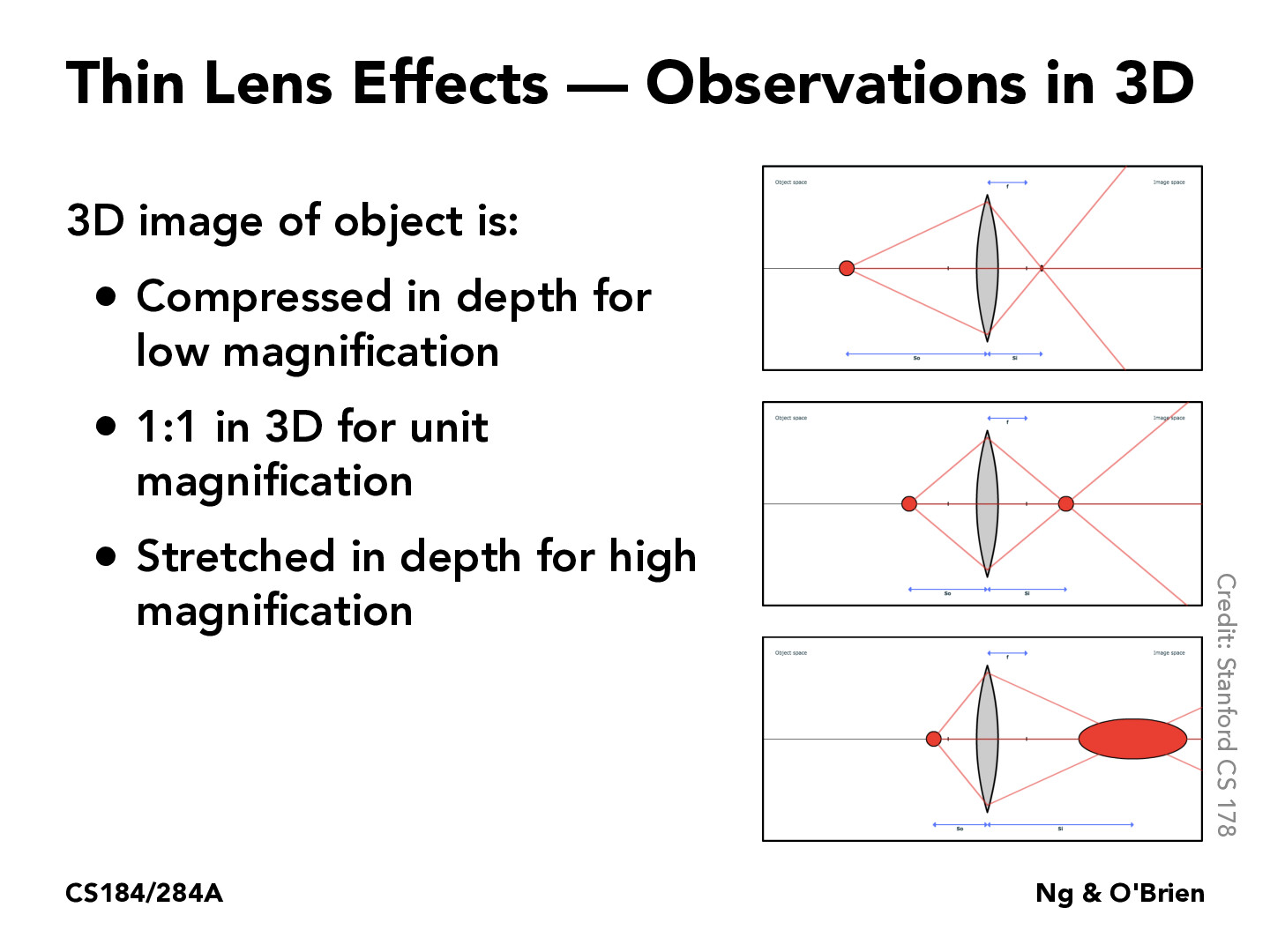Lecture 15: Cameras & Lenses (81)CharlesLiu02

Does this mean that when rays converge at a point that is not the focal point, the image is stretched or compressed?LeslieTrue

@CharlesLiu02, not the focal point, but 2 times the focal length away from th lens. Here's the formula: $\frac1u + \frac1v = \frac1f$, where u and v are the distance from the lens to the real object/screen respectively.

You must be enrolled in the course to comment